# Welcome to Physics 102 Electricity Magnetism forces that

• Slides: 21Welcome to Physics 102! • Electricity & Magnetism (forces that hold atoms & molecules together, living cells) • Light • Atomic & Nuclear Physics Phys. 102, Lecture 1, Slide 1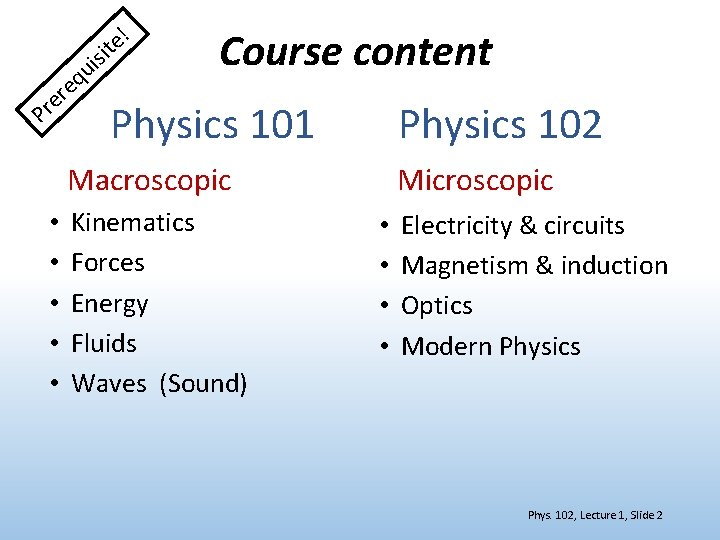! e t i is u q e r re P Course content Physics 101 Physics 102 Macroscopic • • • Kinematics Forces Energy Fluids Waves (Sound) Microscopic • • Electricity & circuits Magnetism & induction Optics Modern Physics Phys. 102, Lecture 1, Slide 2Meet the course directors • Lecturer: Research: Office Hours: • Discussion coordinator: • Lab & exam coordinator: Phys. 102, Lecture 1, Slide 3When emailing me: • Email must be sent from @illinois. edu • Subject line should begin with “PHYS 102 question: ” • Message should contain: your full name, Net. ID, discussion section, TA name • Questions about physics: Do not use email, use office hours (see course website) • Before emailing: Verify information is not already on the course website The course directors reserve the right to penalize your HW score if you ask questions via email that are answered on the website Phys. 102, Lecture 1, Slide 4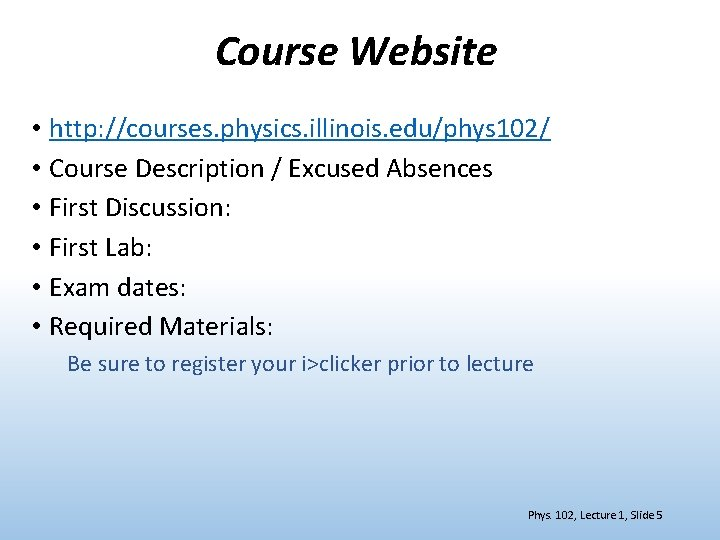Course Website • http: //courses. physics. illinois. edu/phys 102/ • Course Description / Excused Absences • First Discussion: • First Lab: • Exam dates: • Required Materials: Be sure to register your i>clicker prior to lecture Phys. 102, Lecture 1, Slide 5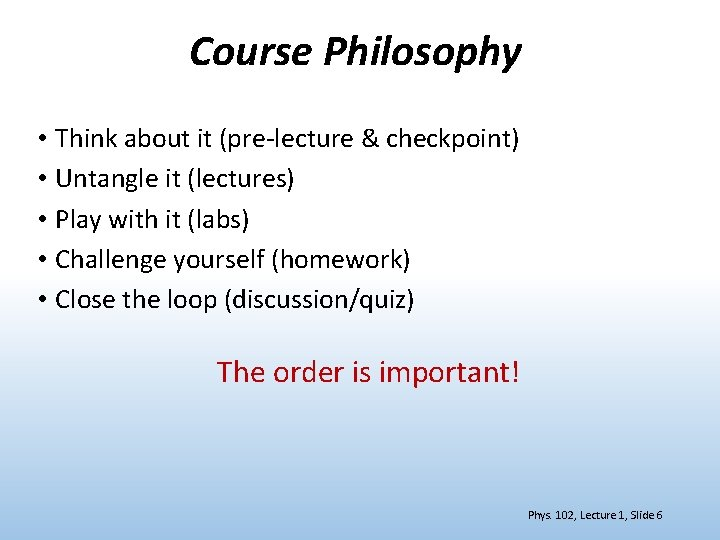Course Philosophy • Think about it (pre-lecture & checkpoint) • Untangle it (lectures) • Play with it (labs) • Challenge yourself (homework) • Close the loop (discussion/quiz) The order is important! Phys. 102, Lecture 1, Slide 6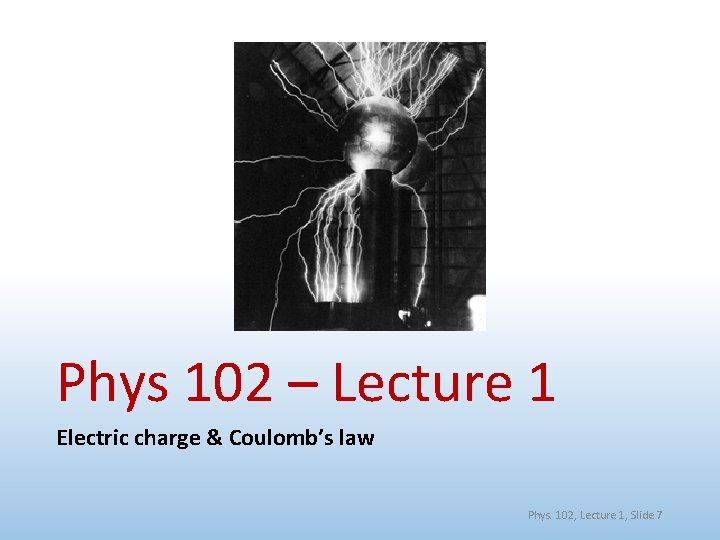Phys 102 – Lecture 1 Electric charge & Coulomb’s law Phys. 102, Lecture 1, Slide 74 Fundamental forces of Nature Phys. 101 Phys. 102 Gravitational force (solar system, galaxies) Electromagnetic force (atoms, molecules) Strong force (atomic nuclei) Weak force (radioactive decay) < Gravitational weakest < Weak < Electromagnetic Strong strongest Phys. 102, Lecture 1, Slide 8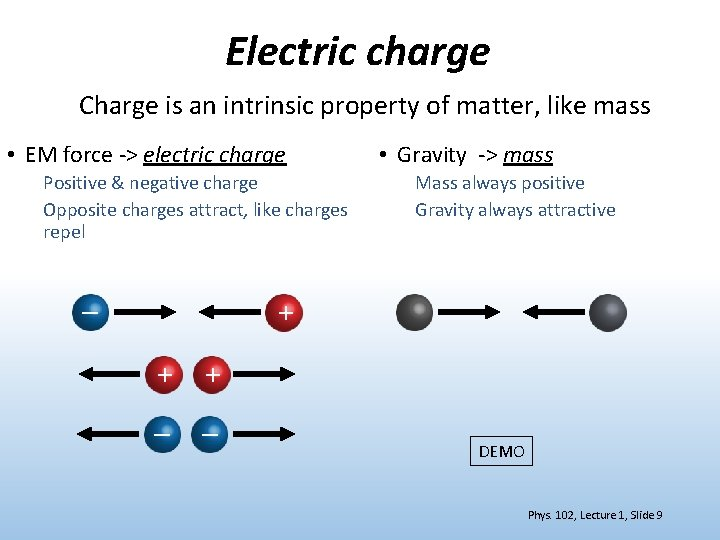Electric charge Charge is an intrinsic property of matter, like mass • EM force -> electric charge Positive & negative charge Opposite charges attract, like charges repel – • Gravity -> mass Mass always positive Gravity always attractive + + + – – DEMO Phys. 102, Lecture 1, Slide 9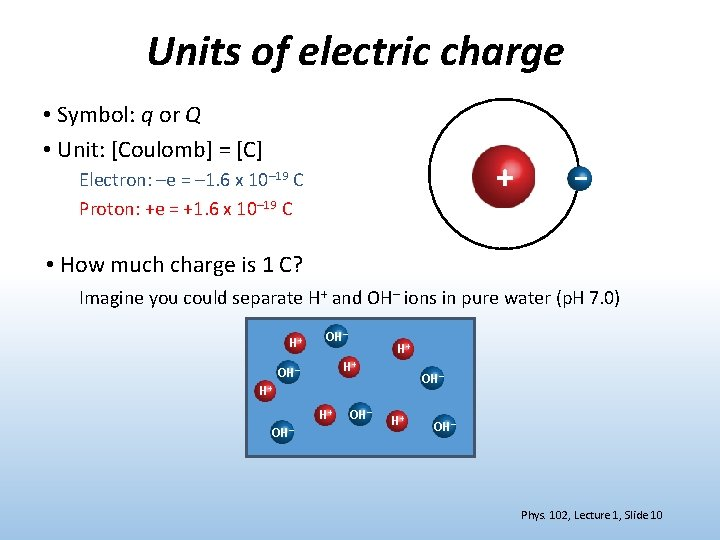Units of electric charge • Symbol: q or Q • Unit: [Coulomb] = [C] + Electron: –e = – 1. 6 x 10– 19 C Proton: +e = +1. 6 x 10– 19 C – • How much charge is 1 C? Imagine you could separate H+ and OH– ions in pure water (p. H 7. 0) H+ OH– H+ H+ OH– OH– H+ OH– Phys. 102, Lecture 1, Slide 10Conductors & insulators Q: How do electrons behave in a perfect conductor? – – Q: How do electrons behave in a perfect insulator? – – – + – – – – + + – + – + Most things are in between perfect conductor / insulator Phys. 102, Lecture 1, Slide 11ACT: Conductors Electrons are placed on a neutral conducting sphere. Which of the following diagrams correctly depicts how the charges are distributed? A. At the bottom B. Spread uniformly C. On the surface – – – – – – DEMO Phys. 102, Lecture 1, Slide 12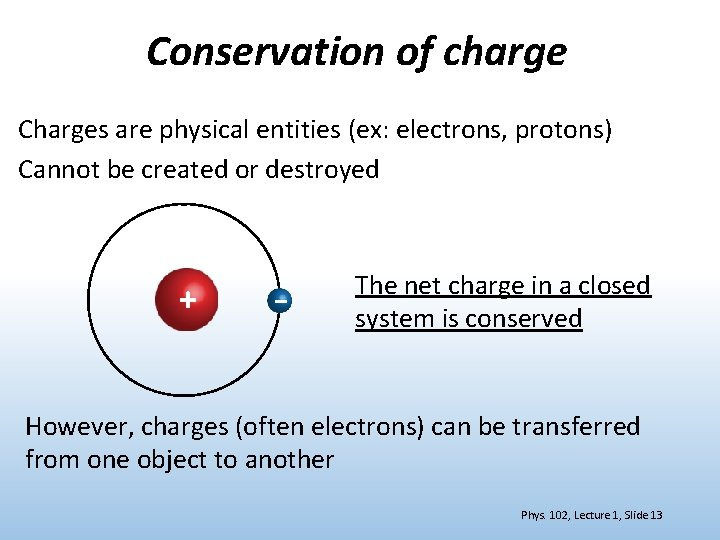Conservation of charge Charges are physical entities (ex: electrons, protons) Cannot be created or destroyed + – The net charge in a closed system is conserved However, charges (often electrons) can be transferred from one object to another Phys. 102, Lecture 1, Slide 13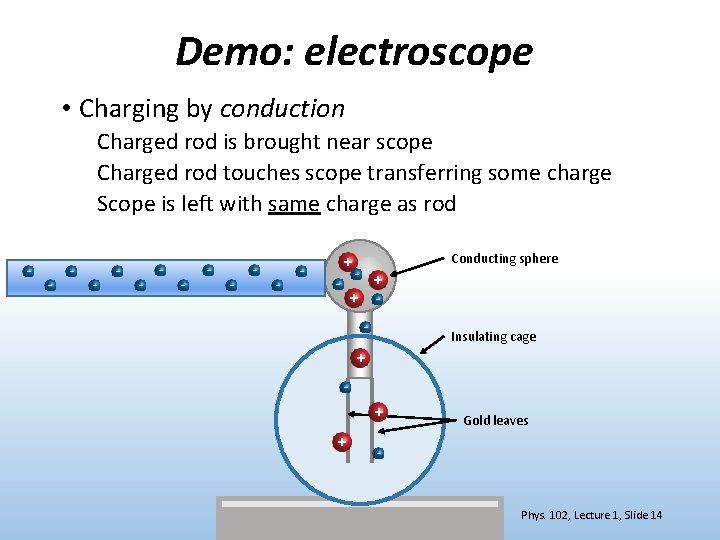Demo: electroscope • Charging by conduction Charged rod is brought near scope Charged rod touches scope transferring some charge Scope is left with same charge as rod – – – Conducting sphere +– – – + + – – Insulating cage + – + + Gold leaves – Phys. 102, Lecture 1, Slide 14Demo: electroscope • Charging by induction Charged rod is brought near scope (but does NOT touch) Scope is briefly grounded allowing charge to flow on (or off) Scope is left with opposite charge as rod – – – +– – + + – Ground wire – + + – – Phys. 102, Lecture 1, Slide 15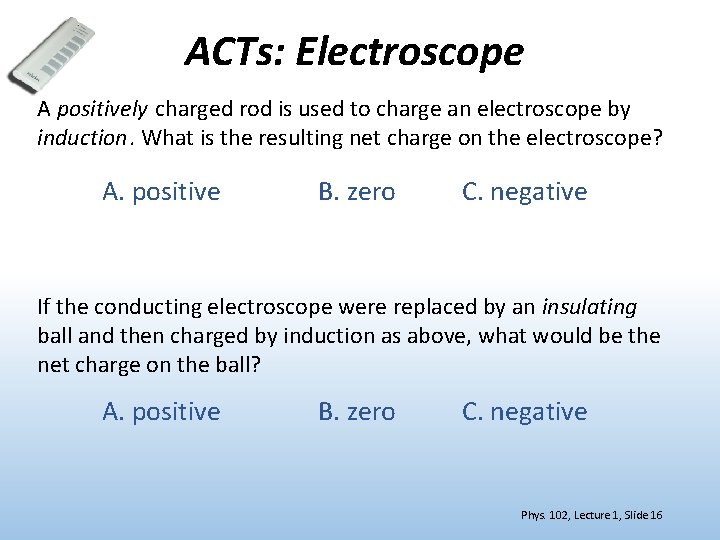ACTs: Electroscope A positively charged rod is used to charge an electroscope by induction. What is the resulting net charge on the electroscope? A. positive B. zero C. negative If the conducting electroscope were replaced by an insulating ball and then charged by induction as above, what would be the net charge on the ball? A. positive B. zero C. negative Phys. 102, Lecture 1, Slide 16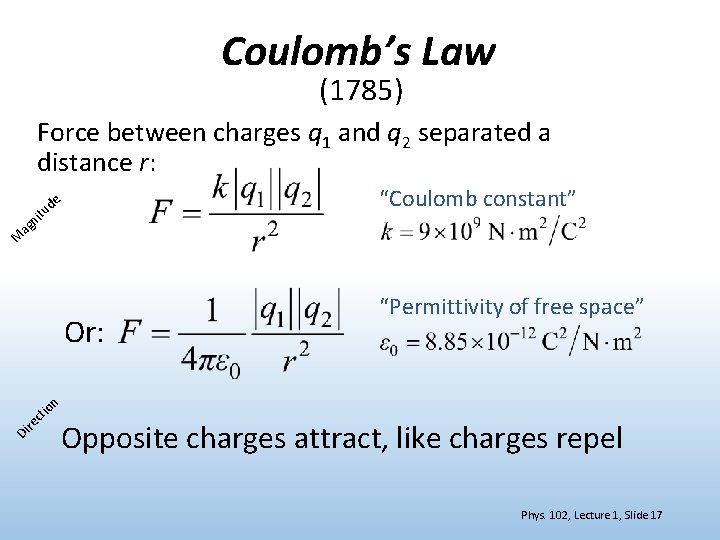Coulomb’s Law (1785) Force between charges q 1 and q 2 separated a distance r: itu n ag “Coulomb constant” de M Or: re Di io ct “Permittivity of free space” n Opposite charges attract, like charges repel Phys. 102, Lecture 1, Slide 17Coulomb’s Law What is the magnitude of the force on the proton due to the electron in hydrogen? Proton qp = 1. 6 x 10– 19 C + – Electron qe = – 1. 6 x 10– 19 C r = 0. 53 x 10– 10 m Compare to gravitational force between them (Phys. 101) Phys. 102, Lecture 1, Slide 18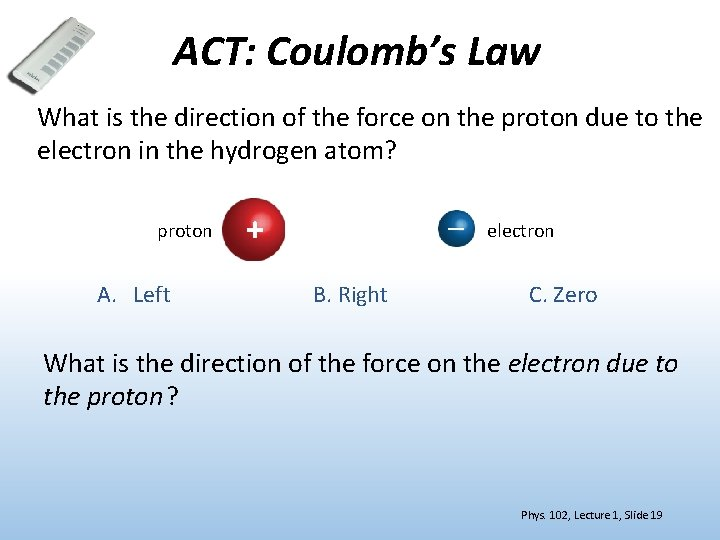ACT: Coulomb’s Law What is the direction of the force on the proton due to the electron in the hydrogen atom? proton A. Left + – B. Right electron C. Zero What is the direction of the force on the electron due to the proton? Phys. 102, Lecture 1, Slide 19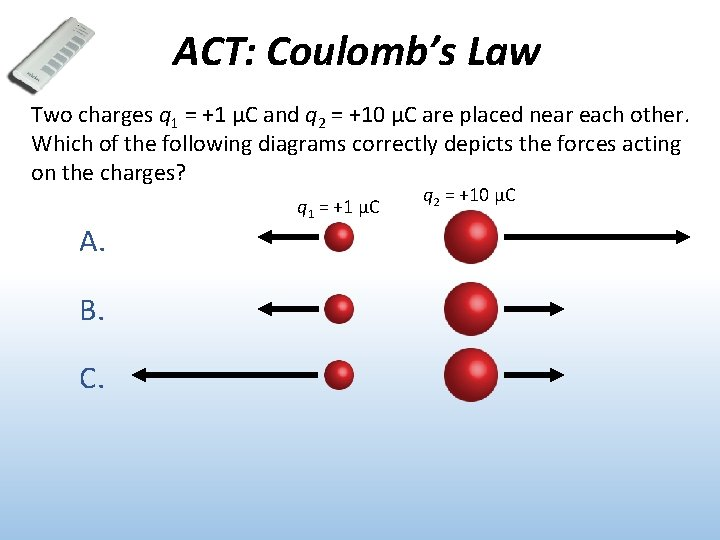ACT: Coulomb’s Law Two charges q 1 = +1 μC and q 2 = +10 μC are placed near each other. Which of the following diagrams correctly depicts the forces acting on the charges? A. B. C. q 1 = +1 μC q 2 = +10 μC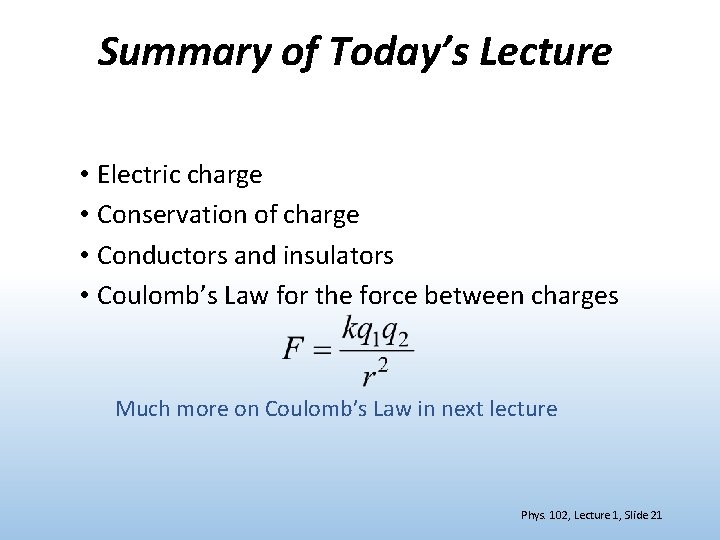Summary of Today’s Lecture • Electric charge • Conservation of charge • Conductors and insulators • Coulomb’s Law for the force between charges Much more on Coulomb’s Law in next lecture Phys. 102, Lecture 1, Slide 21